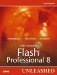# Functions That Return Values

 Currently we are using functions to run repetitive code using parameters, but all the functions are doing is running code. Now let's make them give back some information. To do this, we'll use the return statement. The return statement does two things. First, when the interpreter reaches the return statement, it causes the function to end. Second, it returns the value of an expression assigned to it, but the expression is optional. Here is a generic template: ` function functionName (parameter:ParameterType):ReturnType{ //script to run when function is invoked return expression; } ` This is where the ReturnType option comes in handybecause you can use strict data typing on the return value for debugging purposes. Now let's look at an example of using the return statement to end a function. This example contains a conditional statement, and if the condition is met, the function will end and will not run the remaining code: ` function myFunction (num:Number):Void{ if(num>5){ return; } trace("Num is smaller than 5"); } //Now we invoke the function twice myFunction(6); myFunction(3); //output: Num is smaller than 5 ` Even though the function is run twice, because the conditional statement in the first function is met, the return statement is run and the function is ended. In the second function, the conditional statement is not met, and the trace statement is run. This time, let's use the return statement to return a value back to us based on an expression we apply to the return statement: ` function fullName (fName:String,lName:String):String{ return fName+" "+lName; } //now we set a variable to the function var myName:String = fullName("David", "Vogeleer"); trace(myName); //output: David Vogeleer ` All we did was set the function to a variable, and the return statement returned the value of the expression we assigned to it to the variable. Notice that we set the return value to the data type String, and if the return value is not that data type, you will get a type mismatch error. Using return statements, you can nest functions within functions and even use them as parameters.Macromedia Flash Professional 8 Unleashed
ISBN: 0672327619
EAN: 2147483647
Year: 2005
Pages: 319